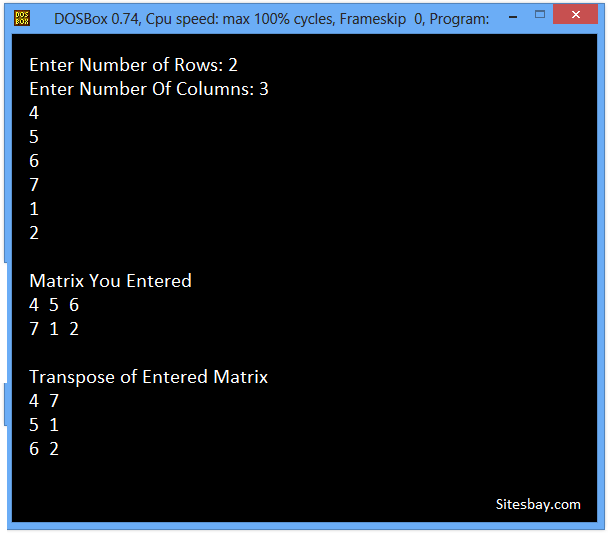# Transpose Matrix Program in C++

## C++ Program to Transpose Matrix

To transpose any matrix in C++ Programming language, you have to first ask to the user to enter the matrix and replace row by column and column by row to transpose that matrix, then display the transpose of the matrix on the screen as shown here in the following C++ program.

### Examples to C++ Program to Transpose Matrix

The transpose of this matrix is shown below: Rows and columns are interchanged, rows of original matrix becomes column in transpose and columns of original matrix becomes rows in transpose.

## Example

```----------------
| 1  | 2  | 3  |
| 4  | 5  | 6  |
| 7  | 8  | 9  |
| 10 | 11 | 12 |
----------------
```

## Example

```---------------------
| 1  | 4  | 7  | 10 |
| 2  | 5  | 8  | 11 |
| 3  | 6  | 9  | 12 |
---------------------
```

To understand below example, you have must knowledge of following C++ programming topics; String in C++, for looping statements we need know For Loop in C++ and Array in C++.

## C++ Program to Transpose Matrix

```#include<iostream.h>
#include<conio.h>

void main()
{

int matrix,transpose_matrix;
int i,j,rows,cols;
clrscr();
// Taking Input In Array

cout<<"Enter Number of Rows: ";
cin>>rows;

cout<<"Enter Number Of Columns: ";
cin>>cols;
for( i=0;i<rows;i++){
for( j=0;j<cols;j++)
{
cin>>matrix[i][j];
}
}

cout<<"\n Matrix You Entered\n";

for( i=0;i<rows;i++){
for( j=0;j<cols;j++)
{
cout<<matrix[i][j]<<"     ";
}
cout<<endl;
}

// Calculating Transpose of Matrix
cout<<"\n\n\nTranspose of Entered Matrix\n";
for( i=0;i<rows;i++){
for( j=0;j<cols;j++)
{
transpose_matrix[j][i]=matrix[i][j];
}
cout<<endl;
}

//Displaying Final Resultant Array
for( i=0;i<cols;i++){
for( j=0;j<rows;j++)
{
cout<<transpose_matrix[i][j]<<"    ";
}
cout<<endl;
}

getch();
}
```

## Output

```Enter Number of Rows: 2
Enter Number Of Columns: 3
4
5
6
7
1
2

Matrix You Entered
4  5  6
7  1  2

Transpose of Entered Matrix
4  7
5  1
6  2
```## Pure VPN Privide Lowest Price VPN Just @ \$1.65. Per Month with Non Detected IP Lowest Price Non Detected IP VPN